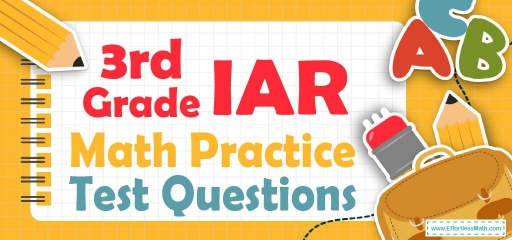# 3rd Grade IAR Math Practice Test QuestionsIs your 3rd-grade student planning to take the Illinois Assessment of Readiness (IAR) math test? In that case, after studying the concepts of the 3rd-grade IAR math test, 3rd-grade students will need practice resources to measure their math skills.

To solve this problem for them, we have come up with a solution: Here are 10 common IAR practice math questions with their exact answers. These questions are the best and by covering the most important mathematical concepts of the IAR test, they will increase the score of your 3rd-grade student in the IAR test.

The Absolute Best Book to Ace the 3rd Grade IAR Math Test

## 10 Sample 3rd Grade IAR Math Practice Questions

1- Which number correctly completes the subtraction sentence
$$8000 – 658 =$$ ___________ ?

A. $$7342$$

B. $$7452$$

C. $$742$$

D. $$7458$$

2- Jason packs $$14$$ boxes with flashcards. Each box holds $$40$$ flashcards. How many flashcards Jason can pack into these boxes?

A. $$56$$

B. $$480$$

C. $$540$$

D. $$560$$

3- Which of the following statements describes the number $$24,589$$?

A. The sum of two thousand, $$4$$ thousand, five hundred, eighty tens, and nine ones

B. The sum of forty thousand, $$2$$ thousand, five hundred, eight tens, and nine ones

C. The sum of twenty thousand, $$4$$ thousand, fifty hundred, eighty tens, and nine ones

D. The sum of twenty thousand, $$4$$ thousand, five hundred, eight tens, and nine ones

4- What is the value of “A” in the following equation?
$$21 + A + 9 = 44$$

A. $$10$$

B. $$12$$

C. $$14$$

D. $$20$$

5- Mr. smith usually eats four meals a day. How many meals does he eat in a week?

A. $$21$$

B. $$24$$

C. $$28$$

D. $$30$$

6- What is the value of $$A$$ in the equation $$72 ÷ A = 8$$

A. $$2$$

B. $$6$$

C. $$7$$

D. $$9$$

7- Use the models below to answer the question.

Which statement about the models is true?

A. Each shows the same fraction because they are the same size.

B. Each shows a different fraction because they are different shapes.

C. Each shows the same fraction because they both have $$3$$ sections shaded.

D. Each shows a different fraction because they both have $$3$$ shaded sections but a different number of total sections.

8- To what number is the arrow pointing?

A. $$36$$

B. $$38$$

C. $$30$$

D. $$42$$

9- Emily has $$108$$ stickers and she wants to give them to nine of her closest friends. If she gives them all an equal number of stickers, how many stickers will each of Emily’s friends receive? ___________

10- The following models are the same size and each is divided into equal parts.
The models can be used to write two fractions.

Based on the models, which of the following statements is true?

A. $$\frac{2}{8}$$ is bigger than $$\frac{4}{16}$$.

B. $$\frac{2}{8}$$ is smaller than $$\frac{4}{16}$$.

C. $$\frac{2}{8}$$ is equal to $$\frac{4}{16}$$.

D. We cannot compare these two fractions only by using the models.

Best 3rd Grade IAR Math Prep Resource

1- A
$$8,000 – 658 = 7,342$$

2- D
$$14 × 40 = 560$$

3- D
$$24,589$$ is the sum of $$20,000; 4,000; 500; 80;$$ and $$9$$.

4- C
$$A = 44 – (21 + 9)$$
$$A = 14$$

5- C
In $$1$$ day $$4$$ meals so $$4 × 7 = 28$$ meals a week.

6- D
$$A = 72 ÷ 8$$
$$A= 9$$

7- D
The model for the first fraction is divided into 6 equal parts. We shade 3_6 to show the same amount as 1_2. The model for the second fraction is divided into 8 equal parts. We shade 3_ 8 that it shows these two models are different fractions.

8- C
The arrow shows exactly the middle of two numbers $$25$$ and $$35$$, so the answer is $$30$$.

9- 12
$$108 ÷ 9 = 12$$

10- C
The model for the first fraction is divided into $$8$$ equal parts. We shade 2_ 8 to show the same amount as 1_ 4. The model for the second fraction is divided into $$16$$ equal parts. We shade 4_ 16 to show the same amount as 1_ 4.

The Best Books to Ace the 3rd Grade IAR Math Test

### What people say about "3rd Grade IAR Math Practice Test Questions - Effortless Math: We Help Students Learn to LOVE Mathematics"?

No one replied yet.

X
30% OFF

Limited time only!

Save Over 30%

SAVE $5 It was$16.99 now it is \$11.99